# 初见

## 简单的例子–戴帽子问题

Hat rule: a student will wear the hat in the following class if one or the other—but not both—of the two classmates sitting immediately on her left and on her right has the hat in the current class (let us say that if nobody wears the hat, then a hat is out of fashion; but if both neighbors wear it, it is too popular to be trendy).

class CA
{
public:
void addElement(bool);
void changeElement(int, bool);
void simulate();
private:
vector<bool> v;
};

void CA::addElement(bool hat)
{
v.push_back(hat);
}

void CA::changeElement(int index, bool hat)
{
v[index] = hat;
}

void CA::simulate()
{
int counter = 50;
while (counter)
{
vector<bool> buf(v);

if (buf == 1)
v = 1;
else
v = 0;

for (int i = 1; i < buf.size() - 1; i++)
{
if (buf[i - 1] ^ buf[i + 1])
{
v[i] = 1;
}
else
{
v[i] = 0;
}
}
if (buf[buf.size() - 2] == 1)
v[buf.size() - 1] = 1;
else
v[buf.size() - 1] = 0;

for (int i = 0; i < v.size() - 1; i++)
if (v[i])
cout << v[i] << " ";
else
cout << "  ";
if (v[v.size() - 1])
cout << v[v.size() - 1] << endl;
else
cout << endl;
counter--;
}
}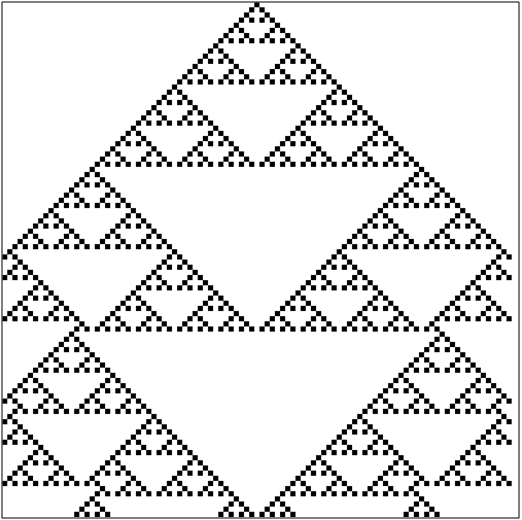戴帽子的情况

# 基本理论

## 四个特性

1. Discrete n-dimensional lattice of cells: We can have one-dimensional, two-dimensional, … , n-dimensional CA. The atomic components of the lattice can be differently shaped: for example, a 2D lattice can be composed of triangles, squares, or hexagons. Usually homogeneity is assumed: all cells are qualitatively identical.

2. Discrete states: At each discrete time step, each cell is in one and only one state, σ ∈ Σ, Σ being a set of states having finite cardinality |Σ| = k.

3. Local interactions: Each cell’s behavior depends only on what happens within its local neighborhood of cells (which may or may not include the cell itself). Lattices with the same basic topology may have different definitions of neighborhood, as we will see below. It is crucial, however, that “actions at a distance” not be allowed.

4. Discrete dynamics: At each time step, each cell updates its current state according to a deterministic transition function φ: Σn → Σ mapping neighborhood configurations (n-tuples of states of Σ) to Σ. It is also usually, though not necessarily, assumed that (i) the update is synchronous, and (ii) φ takes as input at time step t the neighborhood states at the immediately previous time step t − 1.

1. 1-dimensional lattice of square cells on a line.

2. Σ = {1, 0} (1 = black or hat on, 0 = white or hat off), so |Σ| = 2.

3. Each cell’s neighborhood is composed by the two nearest cells. If we index the cells by the integers, so that ci is cell number i, then the neighborhood of ci is N(ci) = <ci − 1, ci + 1>.

4. The transition rule φ is simply stated: At each time step t, a cell state is 1 if exactly one of the neighboring cells was 1 at t − 1, 0 otherwise.

## Wolfram的初等元胞自动机

Wolfram，这个人是谁我不太了解，不过有一个搜索引擎，叫做WolframAlpha，前段时间非常火，它跟元胞自动机有啥关系不知道，不过听说WolframAlpha的加载页面就是元胞自动机的样子。也不懂是什么意思。

### 转换函数分类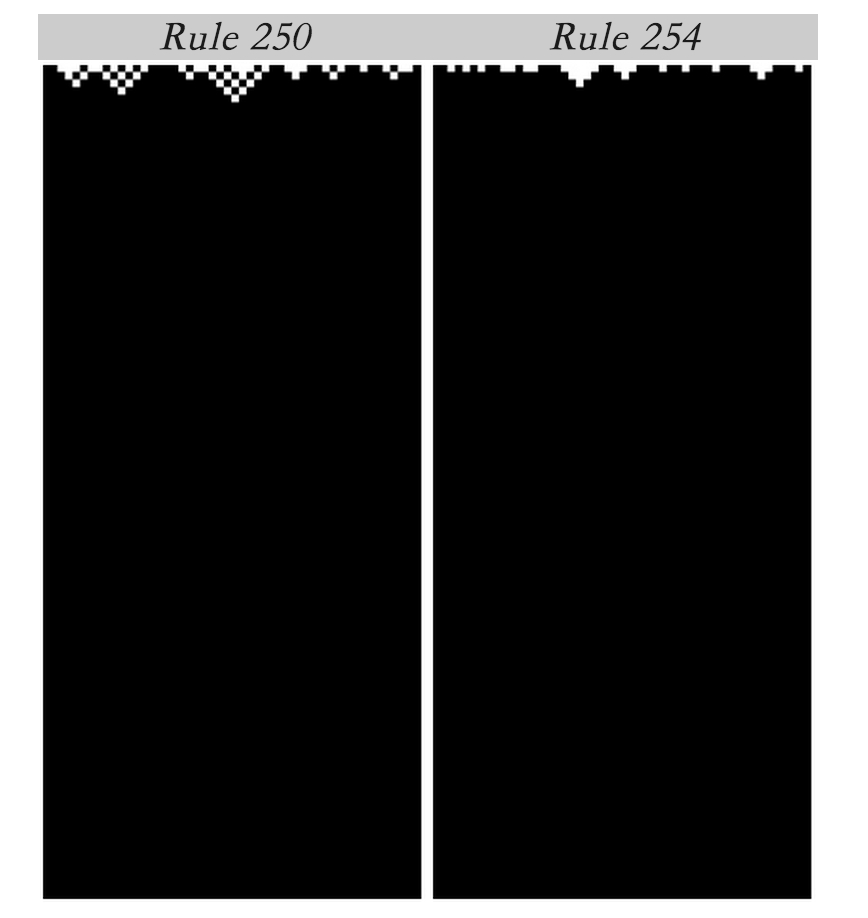class 1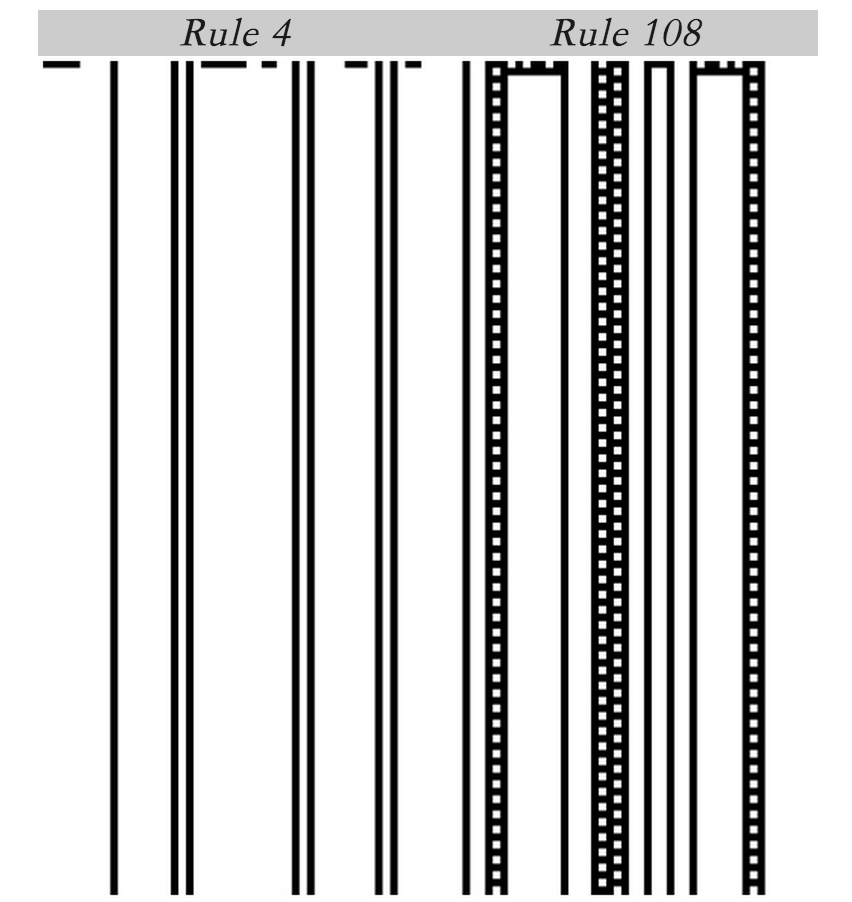class 2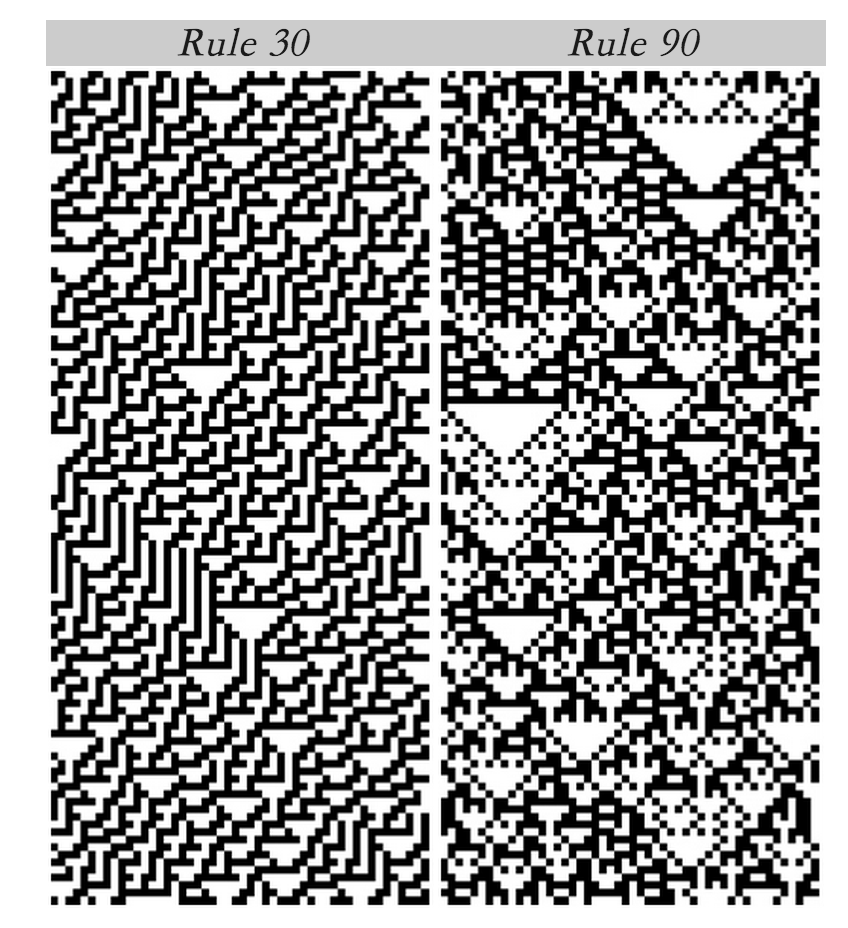class 3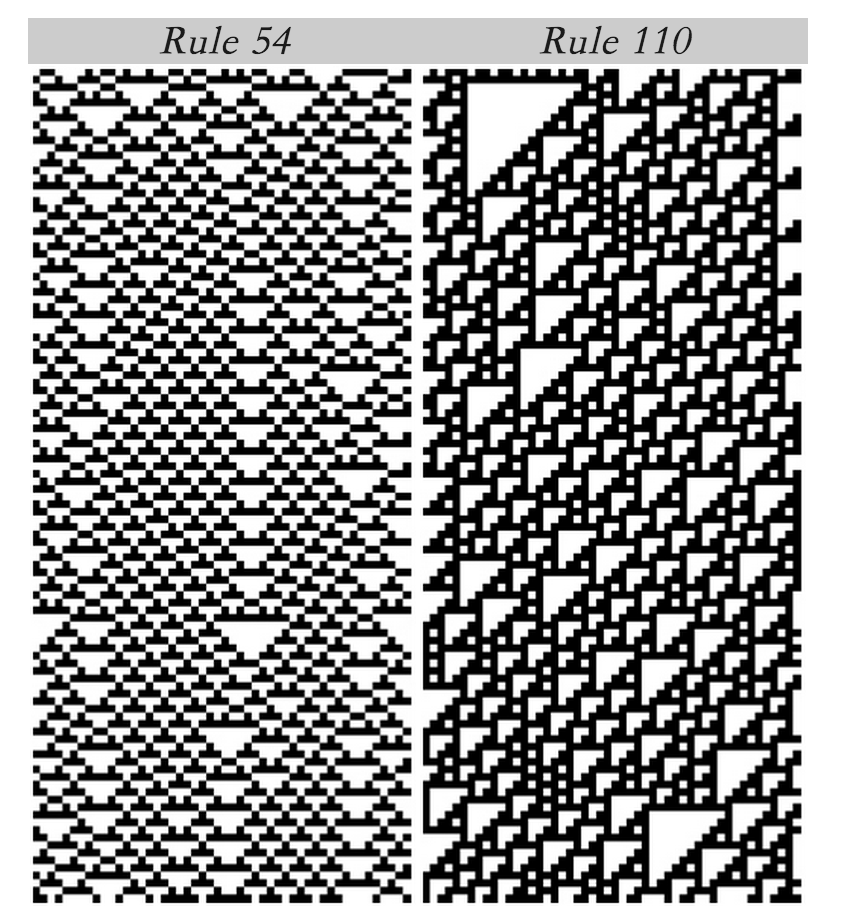class 4

### 第184号规则–车辆行驶规则

111->1
110->0
101->1
100->1
011->1
010->0
001->0
000->0